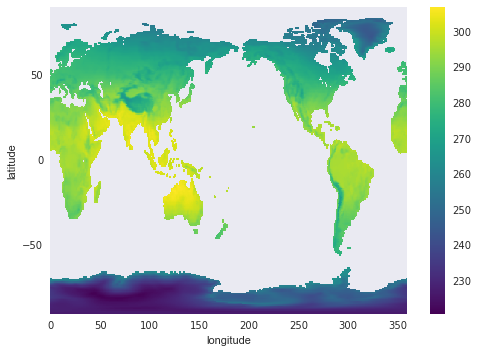## Overview

Teaching: 10 min
Exercises: 5 min
Questions
• What is masking and how can it be used to analyze portions of a dataset

Objectives
• Learn the concepts of masking with xarray.

## Masking with where:

So far we have used indexing to return subsets of the original. The subset array shape will be different from the original. However, we often want to retain the array shape and mask out some observations. There are applications here in remote sensing, land cover modeling, etc.

Suppose we need to determine which grid cells had temperatures > 20 deg C on June 21, 1984? We will use where() for this selection:

``````ds.sel(time="1984-06-21")['t2m'].where(ds.t2m > 293.15).plot()
``````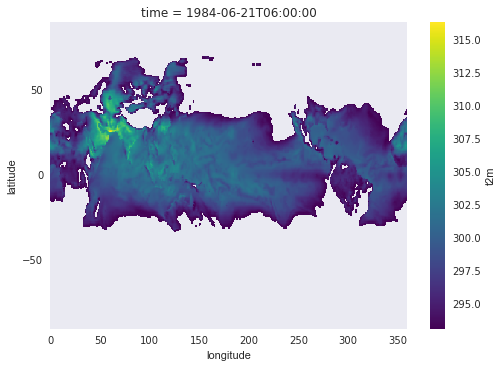Another common Earth science application is to create land cover masks. Let’s use the sea surface temperature field (sst) to build a land and ocean mask. We’ll assign land a value of 1, and ocean a value of 2 (arbitrary). Note that the sst field currently has NaN for all land surfaces:

``````ds.sst.isel(time=0).plot()
``````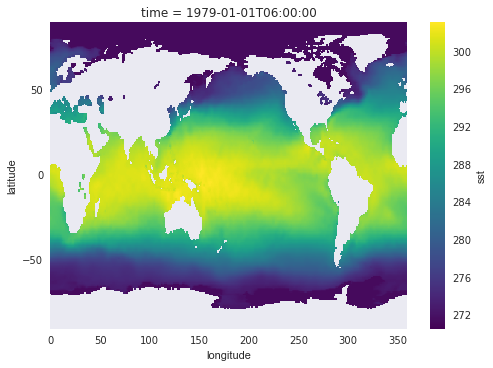### Buliding the mask:

Here we’ll use some lower-level numpy commands to build the mask (and we’ll need to import the numpy library). The mask number depends on whether the cells are finite or NaN:

``````import numpy as np
mask_ocean = 2 * np.ones((ds.dims['latitude'], ds.dims['longitude'])) * np.isfinite(ds.sst.isel(time=0))
mask_land = 1 * np.ones((ds.dims['latitude'], ds.dims['longitude'])) * np.isnan(ds.sst.isel(time=0))
``````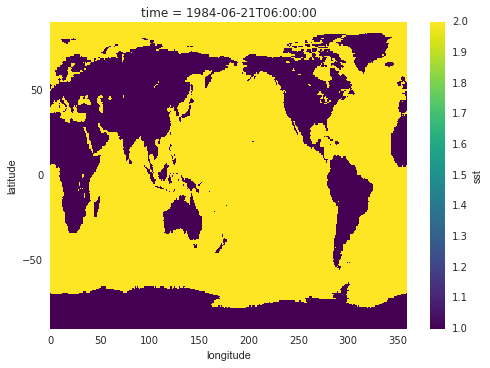### Mask as Coordinates

We can keep the mask as a separate array entity, or, if we are using it routinely, there are advantages to adding it as a coordinate to the `DataArray`:

``````ds.coords['mask'] = (('latitude', 'longitude'), mask_array)
ds
``````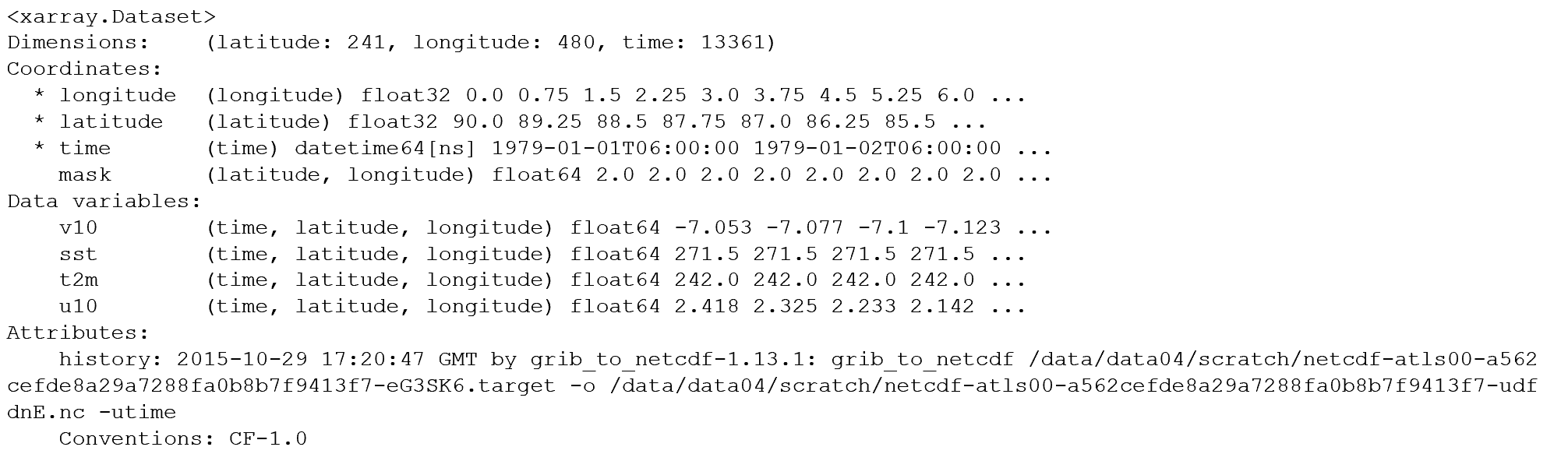Now that the mask is integrated into the coordinates, we can easily apply the mask using `where()`. We can integrate this with statistical functions operating on the array:

``````with ProgressBar():